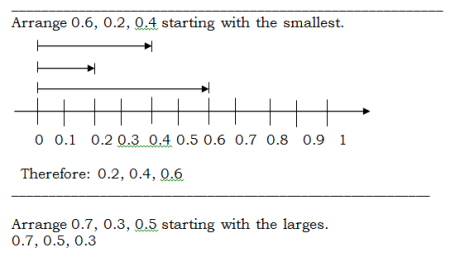• No products in the cart.

# MTH/P/4: FRACTIONS

##### This a mathematics unit studied in primary four and covers the basics of fractions.

Definition of Fractions:

A fraction is part of a whole.

Numerator and denominator

The numerator is a digit on top or above the bar.

The denominator is a digit below or under the bar.

e.g.    2        à      Numerator

3        à      Denominator

The numerator tells us the number of parts we have taken while the denominator tells us the number of parts of equal size the object is divided int.

 Fraction Numerator Denominator 13 1 3 25 2 5

_______________________________

LESSON  TWO.Number of parts

=      3/8

Number of parts

=       5/8

Example:

Write the shaded fraction in the figure.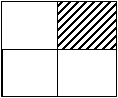Solution:     The figure must be divided

In to equal part  =       1/4Shade 1/3

1
of 6 parts

3

=        6 parts ÷ 3 x 1

=        2 parts x 1

=        2 parts

LESSON  THREE.

TYPES OF FRACTION

Proper fractions

A fraction  whose  numerator is less than its denominator.

e.g. ____2/5_ 1/6__ 12/34_____100/126 etc__________________________________________

Improper fractions

It is a fraction whose numerator is bigger than the denominator.

e.g.    ,  ,   e.t.c.

Mixed fractions

Is a fraction with a whole number and a common or proper fraction.

e.g.,   1/2,   10 1/8, 4/5,   e.t.c.

LESSON  FOUR.

Changing mixed fractions into improper fractions:

Mixed fraction:    4

4 –      Whole number

2 –     Numerator

3 –     Denominator

(Denominator x whole number) + Numerator

Denominator

(d x w) + n            =        (3 x 4) + 2

d                                    3

=        12 + 2

3

=          14

3

Change 5  into an improper fraction.

5  =        (3 X 5) + 2

3

=        15 + 2

3

=           17

3

LESSON  FIVE.

Changing Improper fractions into mixed  fractions.

Examples:

1. Change into a mixed fraction.

3÷ 2 = 1 rem. 1

Therefore,  = 1 1/2

Equivalent fractions

These are fractions which have the same value when simplified.

e.g.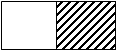½                       =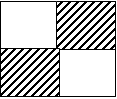2/4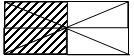4/8

N.B. All the shaded parts are equal.

.:   The  equivalent fractions for ½ are 2/4, 4/8, e.t.c.

LESSON  ONE.

Finding equivalent fractions by calculation

List down 3 equivalent fractions of 1/3

1. 1/3 =       1/3 x  2/2 =        2/6

1/3    =       1/3 x  3/3 =        3/9

1/3        =       1/3x 4/4  =        4/12

1/3        =       1/3 x 5/5  =        5/15

1. 2/5 =       2/5 x  2/2 =        4/10

2/4        =        2/4 x 3/3  =        6/12

2/4        =       2/4 x 4/4  =        8/16

2/5        =       2/5 x 5/5 =        10/25

LESSON  TWO.

Finding the missing number

1. ½ = /6

=  6  ÷ 2

=        3

=   1 x 3

=       3

1. 2/5 =   10/x

=    10  ÷ 2

=    5

=    5 x 5

=   25

LESSON  THREE.

Reducing fractions to their lowest terms.

Equivalent fractions can also be made by dividing the numerator by the same number (G.C.F.).

N.B.   When there is no whole number which can be divided

exactly into the numerator and denominator then the

fraction is in its lowest terms.

1. Reduce 4/6 to its lowest terms.

4        ÷        2        =        2

6        ÷        3                 3

1. Reduce 9/18 to its simplest form.

9        ÷        9        =        1

18      ÷        9                   2

LESSON  FOUR.

Comparing fractions

Which is greater; ½ or 1/3?

Rename       1/2    =        2/4    =        3/6

1/3    =        2/6

1/2    is greater.

Use >, < or = to complete

2 /3  _________  4/6

2/3  =  4/6  =  6/9

4/6  =  8/12  = 12/18

2/3  =  4/6

LESSON  FIVE.

Ordering fractions

Arrange the following fractions starting from the smallest:

1/3, 1/2  , 1/4

Rename:      1/3    =        2/6  =  3/9  =  4/12  =  5/15

1/2    =        2/4  =  3/6  =  4/8  =  6/12

1/4    =        2/8  =  3/12  =  4/16  =  5/20

The order is: 1/4, 1/3, 1/2

LESSON  ONE AND TWO.

Addition of fractions with the same denominators:

Addition of fractions with the same denomination

1. 1/5 + 2/5 =        42/5  =        3/5
1. 3/7 + 2/7 + 2/7 =        3 + 2 + 2     =        7  = 1                                                                                                                   7                                                     7                       7

Addition of fractions with different denominators

Rename: =        1/2 =  2/4  = 3/6  =      4/8

=        1/3  = 2/6 = 3/9  =       4/12

½ + 1/3      =        3/6 + 2/6   =        3+2    = 5/6

6

1. 2/3 + 1/4

Rename: 2/3  =  4/6  =  6/9  =  8/12  =  10/15

¼  =    2/8   = 3/12   = 3/12  =  4/16  =  5/12

2/3 + 1/4=      8/12 + 3/12         8 + 3  =        11

12               12

LESSON THREE

1. 6 2/7 + 1 3/7 =        (6 + 1) + 2/7  +  3/7

=        7  +  2+3

7

=        7 + 5/7

=        7 5/7

LESSON  FOUR.

Subtraction of fractions with the same denominators

1. 5/6 –  1/6       =        5 – 1  =        4

6               6

1. Subtract: 4 2/5  –  2 1/5

=        (4 – 2) + (2/5 – 1/5)

=        2 + 2 – 1

5

Subtract fractions with different denominators

Subtract:    1/2 – 1/3

Rename:      1/2  – 1/3  =  3/6  =  4/8  =  5/10  =  6/12

1/3  =  2/6  =  3/9  =  4/12  =  5/15  = 6/18

½-1/3         =        3/6  –  2/6  = 3-2

6

=        1/6

LESSON  FIVE.

Subtracting a fraction from a whole

1. 1 –  1/2

2/2 – 1/2   =   2-1    = 1/2

2

1. 1 –  3/12

12/12  –  3/12 = 12 – 3       =9/12

12

1. John at ¼ of a cake. What fraction of the cake is left?

1 – ¼ =        4/4 – ¼

=        4 – 1 =  3/4

4

Subtractions of mixed fractions.

1. 7 2/5 _ 2 1/5

= (7_ 2 ) + ( 2/5 _ 1/5 )

= 5 + 1/5

=5 1/5

WEEK  FIVE

LESSON  ONE

Multiplication of  fractions

Multiplication of a fraction by a whole (parts of a group)

1. What is ½ of
2. 2/3 of 6 oranges= 4 oranges

OR:    2/3  x 6   =  (6  ÷ 3)  x  2          2/3 x 6

=        2 x 2                                        2 x 6 = 12 = 4

=        4 oranges                                    3        3

1. 4/5 x 20 boys

(20 ÷ 5)  x 4

4 x 4

16 boys

Multiplying a fraction by a fraction:

1. 1/3 x 2/4 =        1 x 2  =        2/12

3 x 4

1. ½ x 1/3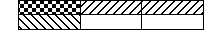Number of parts shaded twice      =        1/6

OR:        ½ x 1/3      =        n x n

d x d

=        1 x 1  =        1/6

2 x 3

LESSON TWO

Division of fractions

Divide a whole by a fraction

Example:    3 ÷ 1/3       (How many thirds are on 3 wholes)++=        9 thirds

3 ÷  1/3      =        9

4 ÷ 1/2       (How many halves  are in 4 wholes)= 8

4 ÷ ½  = 8

LESSON THREE

Application of fractions

1. There are 24 boys in a class. If 2/3 of them play football, how many boys play football?

2/3 of 24    =        2/3 x 24

=        (24 ÷ 3) x 2

=        8 x 2

=        16 boys

1. There are 40 pupils in P.4. 2/5 of them are boys and the rest are girls.
2. Find the fraction for girls.

1 – 2/5        =        5/5 – 2/5

=        3/5

1. How many boys are in the class?

2/5 of 40    =        2/5 x 40

=        2 x 8

=        16 boys

1. How many girls are in the class?

4 0                                           3/5 of 40

–   1 6                                  =        3/5 x 40

2 4     girls            OR:    =        3 x 8  = 24 girls

LESSON  FOUR.

DECIMALS

Changing common fractions into decimals

1. Change 2/10 into a decimal.

0.2

2/10      =        10|2

0 x 10 = – 0

2 0        =        0.2

2 x 10 = – 2 0

– –

1. Change 3/10 into a decimal

0.3

3/10      =        10|3

0 x 10 = – 0

3 0        =        0.3

3 x 10 = –3 0

1. Change 2/5 into a decimal.

0.4

2/5        =          5|2

0 x 5  = –  0

2 0        =        0.4

4 x 5  = – 2 0                                   – –

LESSON  FIVE.

LESSON  ONE.

Expressing mixed fractions as decimals

1. Change 1 7/10 to a decimal

=        1 + 7/10

=        1 + 0.7

=        1.0

+ 0.7

1.7

1. Change 2 4/10 to a decimal

=        2 + 4/10

=        2 + 0.4

=        2.0

+ 0.7

2.4

LESSON  TWO

Expressing decimals as mixed fractions

1. Change 1.5 to a common fraction

1.5         =        (1 x 1) + (5 x 1/10)

=        1 + 5/10

=        5/10

1. Write 12.9 as a common fraction

12.9       =        (1 x 10) + (2 x 1) + (9 x 1/10)

=        0 + 2 +  9/10

=        2 + 9/10

=        2  9/10

LESSON  THREE.

=   1.3

+ 2.6

3.9

1. Find the sum of 1.4 and 2.8

=   1.4

+2.8

4.2

1. I ate 0.2 of a cake in the morning, 0.1 in the afternoon and 0.3 in the evening. What fraction did I eat altogether

0.2

0.1

+   0.3

0.6

LESSON  FIVE.

Ordering and comparing decimals

Use >, <, or = to complete

1. 2 < 0.3

2/10   3/10

1. 7 >  0.4

7/10  >  4/10